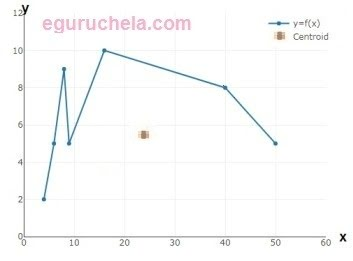# Polygon Centroid Point Calculator

Polygon calculator to calculate centroid point of polygon area for entered values of side of polygon.

 No of sides of polygon :

 X axis centroid position Y axis centroid position

First enter the number of side polygons then system show the required boxes to enter the x, y coordinates.

Press calculate button### Example

Find the centroid for polygon having 7 sides as (4, 2) (6, 5) (8, 9) (9, 5) (16, 10) (40, 8) (50, 5)

Calculator will retun the value (24.175, 5.909)